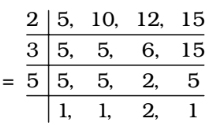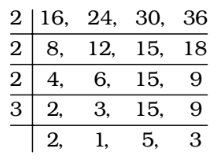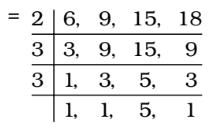## LCM and HCF

#### LCM and HCF

1. What least number must be subtracted from 1936 so that the resulting number when divided by 9, 10 and 15 will leave in each case the same remainder 7 ?

1. LCM of 9, 10 and 15 = 90
⇒ The multiple of 90 are also divisible by 9, 10 or 15.
∴ 21 × 90 = 1890 will be divisible by them.
∴ Now, 1897 will be the number that will give remainder 7.
∴ Required number = 1936 – 1897

##### Correct Option: C

LCM of 9, 10 and 15 = 90
⇒ The multiple of 90 are also divisible by 9, 10 or 15.
∴ 21 × 90 = 1890 will be divisible by them.
∴ Now, 1897 will be the number that will give remainder 7.
∴ Required number = 1936 – 1897 = 39

1. The smallest number, which when divided by 5, 10, 12 and 15, leaves remainder 2 in each case; but when divided by 7 leaves no remainder, is

1. LCM of 5, 10, 12, 15∴ LCM = 2 × 3 × 5 × 2 = 60
∴ Number = 60k + 2
Now, the required number should be divisible by 7.
Now, 60k + 2 = 7 × 8k + 4k + 2
If we put k = 3 , (4k + 2) is equal to 14 which is exactly divisible by 7.

##### Correct Option: B

LCM of 5, 10, 12, 15∴ LCM = 2 × 3 × 5 × 2 = 60
∴ Number = 60k + 2
Now, the required number should be divisible by 7.
Now, 60k + 2 = 7 × 8k + 4k + 2
If we put k = 3 , (4k + 2) is equal to 14 which is exactly divisible by 7.
∴ Required number = 60 × 3 + 2 = 182

1. The largest number of five digits which, when divided by 16, 24, 30, or 36 leaves the same remainder 10 in each case, is :

1. The n digit largest number which when divided by p, q, r leaves remainder ‘a’ will be
Required number = [n – digit largest number – R] + a
where, R is the remainder obtained when n–digit largest number is divided by the L.C.M of p, q, r.
We will find the LCM of 16, 24, 30 and 36.∴ LCM = 2 × 2 × 2 × 3 × 2 × 5 × 3 = 720
The largest number of five digits = 99999
On dividing 99999 by 720, the remainder = 639

##### Correct Option: B

The n digit largest number which when divided by p, q, r leaves remainder ‘a’ will be
Required number = [n – digit largest number – R] + a
where, R is the remainder obtained when n–digit largest number is divided by the L.C.M of p, q, r.
We will find the LCM of 16, 24, 30 and 36.∴ LCM = 2 × 2 × 2 × 3 × 2 × 5 × 3 = 720
The largest number of five digits = 99999
On dividing 99999 by 720, the remainder = 639
∴ The largest five-digit number divisible by 720
= 99999 – 639 = 99360
∴ Required number = 99360 + 10 = 99370

1. The least multiple of 7, which leaves the remainder 4, when divided by any of 6, 9, 15 and 18, is

1. LCM of 6, 9, 15 and 18∴ LCM = 2 × 3 × 3 × 5 = 90
∴ Required number = 90k + 4, which must be a multiple of 7 for some value of k.
For k = 4,

##### Correct Option: D

LCM of 6, 9, 15 and 18∴ LCM = 2 × 3 × 3 × 5 = 90
∴ Required number = 90k + 4, which must be a multiple of 7 for some value of k.
For k = 4,
Required Number = 90 × 4 + 4 = 364, which is exactly divisible by 7.

1. What is the least number which when divided by the numbers 3, 5, 6, 8, 10 and 12 leaves in each case a remainder 2 but when divided by 13 leaves no remainder ?

1. We find LCM of 3, 5, 6, 8, 10 and 12 = 120
∴ Required number = 120q + 2, which is exactly divisible by 13.
Required number = 120q + 2 = 13 × 9q + 3q + 2
Clearly 3q + 2 should be divisible by 13.
For q = 8 , 3q + 2 is divisible by 13.

##### Correct Option: B

We find LCM of 3, 5, 6, 8, 10 and 12 = 120
∴ Required number = 120q + 2, which is exactly divisible by 13.
Required number = 120q + 2 = 13 × 9q + 3q + 2
Clearly 3q + 2 should be divisible by 13.
For q = 8 , 3q + 2 is divisible by 13.
∴ Required number = 120q + 2 = 120 × 8 + 2
Required number = 960 + 2 = 962